American Journal of Civil Engineering
Volume 4, Issue 6, November 2016, Pages: 337-344

Analysis on Difference Between Rock Salt Dissolving Model with and Without Mechanical Effect

Tang Yanchun1, *, Liu Ruilong1, Zhou Hui2

1College of Civil Engineering and Architecture, China Three Gorges University, Yichang City, China

2State Key Laboratory of Geomechanics and Geotechnical Engineering, Institute of Rock and Soil Mechanics, Chinese Academy of Sciences, Wuhan City, China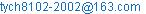(Tang Yanchun)

*Corresponding author

Tang Yanchun, Liu Ruilong, Zhou Hui. Analysis on Difference Between Rock Salt Dissolving Model with and Without Mechanical Effect. American Journal of Civil Engineering. Vol. 4, No. 6, 2016, pp. 337-344. doi: 10.11648/j.ajce.20160406.20

Received: November 9, 2016; Accepted: November 28, 2016; Published: December 1, 2016

Abstract: For the stability of rock salt cavern during the washing process, the research on the relationship between the dissolving mechanism and mechanical effect of rock salt is necessary. Through analyses on rock salt dissolving mechanism, and rock salt dissolving effect with and without mechanical effect, difference between rock salt dissolving model with and without mechanical effect has been studied. It can be concluded that rock salt dissolving mechanism with and without mechanical effect is same, but with mechanical effect, especially after the plastic strain and cracks have been produced, difference between the dissolving effect with and without mechanical effect has been existed; compared with the parameter "diffusion coefficient" D on the dissolving model without mechanical effect, the new conception "equivalent diffusion coefficient" D* has been proposed to describe the macro-dissolving speed of rock salt with mechanical effect under the assumption that dissolving action face has not been changed during the dissolving process. D* is a variable of the equivalent plastic strain and dissolving time; based on rock salt dissolving model without mechanical effect and the new concept D*, rock salt dissolving model with mechanical effect has been built; through analyzing the datum of uniaxial compression meso-mechanical test under the coupled mechanical-dissolving effect, the method to calculate D* has been proposed, and the relationship between axial plastic strain, dissolving time and D* has been obtained. The achieved results can provide a research foundation for the further analyses on coupled mechanical-dissolving mechanism of rock salt.

Keywords: Rock Salt, Dissolving Mechanism, Mechanical Effect, Equivalent Diffusion Coefficient, Axial Plastic Strain

1. Introduction

Because of high security and easy construction, rock salt cavern, which has been called high strategic security energy storage vault, has been applied to underground energy storage broadly on many countries [1,2]. To ensure the sustainable development of economics and society of China, it is necessary to build the strategic energy storage and commercial energy storage over a period of time in the future . On the washing process of building cavern, the stability of rock salt cavern has been mainly controlled by two factors, the stress distribution [4-7] and dissolving characteristics of rock salt on wall rock [8-11]. Some researchers have acquired abundant research results on the washing process and stability of rock salt cavern taking account of the above two factors respectively, but the coupled effect on the above two factors has not been taking into account deficiently.

In fact, during the washing process, interaction between the mechanical effect and dissolving characteristics of rock salt on wall rock of rock salt cavern is existed: on the one hand, because of the mechanical effect on wall rock, cracks have been produced, dissolving characteristics of rock salt on wall rock has been changed, the macro dissolving speed of rock salt has been enlarged; on the other hand, because of the variation of dissolving characteristics, the mechanical property of rock salt and the stability of rock salt cavern has been influenced un-neglectfully. And therefore, the research on the relationship between the dissolving mechanism and mechanical effect of rock salt is necessary on the analysis of washing process and stability of rock salt cavern.

However, the research result about the above problem is inadequate. On this paper, through analyses on rock salt dissolving mechanism, and rock salt dissolving effect with and without mechanical effect, difference between rock salt dissolving model with and without mechanical effect has been studied.

2. Rock Salt Dissolving Mechanism

Actually the rock salt dissolving process is the process of diffusion that the rock salt molecule has been diffused onto the water solution. The sketch of rock salt dissolving process has been shown in Fig. 1. In Fig. 1, the dissolving process of rock salt can be simplified as follow:

The water solution can be assumed to be divided by the boundary layer and the diffusion layer.

The boundary layer which is very thin is adhered to rock salt solid boundary. And the concentration of rock salt solution on the boundary layer can be assumed to a constant that is valued to saturated concentration of rock salt solution.

In the boundary layer, the dynamic balance of the total mass between the dissolution and diffusion process has been maintained.

In the diffusion layer, the diffusion and convection effect for fluid has been observed.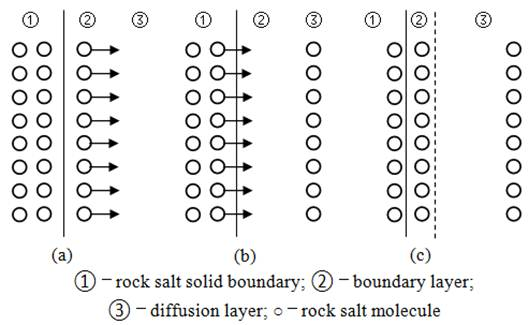Figure 1. Sketch of rock salt dissolving process.

Based on the above assumption, the dissolving process can be divided into three steps as follows:

1)   Because of diffusion effect, the rock salt molecule of the boundary layer has been diffused into the diffusion layer, and the concentration of rock salt solution on the boundary layer has been decreased.

2)   On the solid boundary, rock salt has been dissolved, and the rock salt molecule has been dissolved onto the boundary layer, and then the concentration of rock salt solution in the boundary layer has been reached the value of saturated concentration again.

3)   Because of dissolving process of rock salt on the solid boundary, the solid boundary and the boundary layer have been back to a tiny distance.

3. Rock Salt Dissolving Model Without Mechanical Effect

Actually the rock salt dissolving process is a complex process of hydrodynamics and chemical kinetics [12,13], and so, according with the dynamics analysis of the three-dimensional convective-diffusion process and the basic theory and chemical kinetics of hydromechanics, rock salt dissolving model without mechanical effect can be built.

3.1. Basic Hypothesis of Model

To the convenience on research, the model can be assumed and simplified as follows:

1)   Assumed that the effect on dissolving characteristics causing by anisotropic properties of rock salt, such as crystal direction and lamination, can be ignored.

2)   During the course of dissolving, the interior process and thermodynamics process of chemical reaction can be ignored.

3)   The effect causing by insoluble impurities on rock salt can be ignored.

4)   Assumed that the fluid cannot be compressed.

5)   Assumed that during the course of fluid transport, only the effect of fluid gravity field can be considered.

6)   The effect causing by temperature on dissolving process can be ignored.

3.2. Fluid Transport Equation

According with the basic theory of incompressible hydrodynamics, the transport equations between mass and concentration on rock salt dissolving process have been built.

Continuity equation

For the incompressible fluid, the continuity equation is as follow: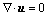(1)

In E. Q. 1,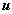is fluid velocity vector.

Kinetic equation

According with Navier-Stokes equation, the fluid kinetic equation can be described as follow: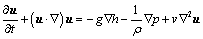(2)

In E. Q. 2, v is dynamic viscosity;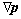is pressure intensity gradient; p is average dynamic pressure intensity of fluid; ρ is density of rock salt solution; h is vertical coordinate, and its direction of positive value is upward.

Convective-Diffusion equation

The rock salt dissolving process can be described by the follow Convective-Diffusion equation: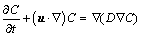(3)

In E. Q. 3, C is molar concentration of rock salt solution; D is diffusion coefficient of rock salt solution.

3.3. Equation of Boundary Shape on Dissolving Process

Fick first scattering law

The diffusion effect can be described by Fick first scattering law as follow: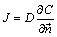(4)

In E. Q. 4, diffusion flux J denotes the mass per unit time and per unit area on the direction which is vertical to the normal of area;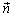is normal direction vector; D denotes the proportionality coefficient that is valued to the mass per unit area and per unit time divided by normal concentration gradient.

Equation of boundary shape

Based on basic hypothesis of model, fluid transport equation and Fick first scattering law, variation of boundary shape on dissolving process can be described as follow.

Along with the normal direction of rock salt boundary, the dissolving sketch on rock salt boundary has been built in Fig. 2. In Fig. 2, the direction X is the tangential direction of rock salt boundary; the direction R is the normal direction of rock salt boundary; infinitesimal body dx×dR which has been marked by the color area in Fig. 2 is unit thickness.Figure 2. Dissolving sketch on rock salt boundary.

For infinitesimal body dx×dR, based on the material equilibrium principle, the equation can be obtained as follow: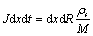(5)

In E. Q. 5, Jdxdt is the mole number of rock salt on the section 1×dx which has been dissolved into solution in time dt;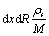is the mole number of rock salt which has been dissolved from the rock salt solid in time dt and the section 1×dx; ρs is density of rock salt solid; M is mole mass of rock salt; dR is thickness of rock salt being dissolved along the normal direction of boundary of rock salt solid in time dt.

Associating with E. Q. 4 and 5, the equation of boundary shape on dissolving process can be expressed as follow: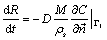(6)

In E. Q. 6, Γ1 is boundary plane of rock salt solid.

3.4. Subsidiary Equation

Based on the characteristics of fluid transport, it can be found that ρ and C are not independent variables. Relationship between ρ and C can be expressed as follow: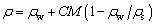(7)

In E. Q. 7, ρw is density of water.

3.5. Solution Conditions

Initial condition

Before dissolving, water solution which contacts with rock salt solid boundary is static. So the initial conditions of model are as follow: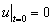(8)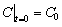(9)

In E. Q. 9, C0 is mole concentration of water solution under the initial state, 0≤C0Cs, Cs is saturated mole concentration of rock salt water solution.

Boundary condition

According with the assumption, rock salt solution on the boundary layer is saturated solution. So the concentration of rock salt solution on the solid boundary plane Γ1 is saturated mole concentration, and the boundary condition can be expressed as follow: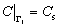(10)

3.6. Expression for Model

In summary, according with continuity equation, kinetic equation, convective-diffusion equation, equation of boundary shape on dissolving process, subsidiary equation and solution conditions of equations, constrained partial differentiation equation system of rock salt dissolving model without mechanical effect can be expressed as follow:(11)

4. Difference Between Rock Salt Dissolving Effect with and Without Mechanical Effect

Rock salt dissolving mechanism with and without mechanical effect is same, but difference between rock salt dissolving effect with and without mechanical effect is existed as follow:

1)   If without mechanical effect, dissolving effect for rock salt can only be happened on the solid boundary, so the dissolving action face is solid boundary surface.

2)   But if with mechanical effect, especially after a number of cracks on the rock salt solid surface have been produced, not only on the solid boundary but also at the interior of the surface cracks, dissolving effect can be happened. So with mechanical effect the dissolving action face is the sum by the rock salt solid boundary surface and all surface cracks.

The cracks on the surface of rock salt sample after uniaxial compression test are as shown in Fig. 3(a), and the shape of surface cracks after dissolved is as shown in Fig. 3(b).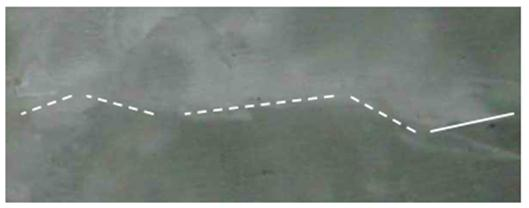(a) Photo of surface crack. Noted that the trend of surface crack has been marked by the white line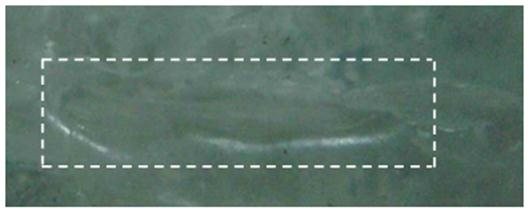(b) Photo of surface crack after dissolved. Noted that the area of surface crack has been marked by white block frame

Figure 3. Variation of rock salt surface crack after dissolved.

In Fig. 3(a) and 3(b), the phenomenon can be observed as follow: after dissolved, the shape of surface crack has been changed obviously, and the jaw opening and the depth of surface crack are larger. This shows that during the dissolving process, the surface area of surface crack has been enlarged and the dissolving action face has been changed, and the influence of rock salt dissolving characteristics causing by mechanical effect can not be ignored.

Based on the above analysis, the results can be obtained: with the increase of plastic strain, the number and size of rock salt crack has been increased, and so the dissolving action face has been enlarged constantly, and then rock salt mass being dissolved at a certain dissolving time which can be equal to the macro-dissolving speed of rock salt has been increased continuously.

Therefore, with mechanical effect, the relationship must be existed in the macro-dissolving speed and plastic strain of rock salt.

5. Rock Salt Dissolving Model with Mechanical Effect

Because the number and size of rock salt surface crack can be hard to count, the area of dissolving action face with mechanical effect can not be computed, so the rock salt dissolving model without mechanical effect can not be directly used to simulate rock salt dissolving characteristics with mechanical effect.

The key points can be noticed as follow:

1)   From the macro point, difference between the macro-dissolving speed with and without mechanical effect which can be equal to the rock salt mass being dissolved at the same dissolving time and the same surface has been existed.

2)   Either with or without mechanical effect, the dissolving effect can be decided by the diffusion effect on the surface, that is mean that the dissolving effect can be controlled by two factors, the area of dissolving action face and diffusion coefficient.

3)   In fact, the area of dissolving action face can be hard to compute, and the value of diffusion coefficient of rock salt solution is a constant.

Based on the above key points, the follow assumptions can be made:

either with or without mechanical effect, during the dissolving process, the area of dissolving action face can be assumed to keep unchanged.

Correspondingly the diffusion coefficient can be assumed to change. Rock salt "diffusion coefficient" D with mechanical effect can be called by "equivalent diffusion coefficient" D*.

Based on the above assumption about the area of dissolving action face and diffusion coefficient, rock salt dissolving model with mechanical effect can be described by rock salt dissolving model without mechanical effect with D*.

The new conception "equivalent diffusion coefficient" D* is proportionality coefficient that is valued to the mass per unit area and per unit time divided by normal concentration gradient under the macro point. D* has been proposed to describe the macro-dissolving speed per unit dissolving area of rock salt with mechanical effect.

Equivalent plastic strain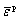can be used to describe the damage degree of rock salt with mechanical effect, and under the same stress-strain condition, the value of macro-dissolving speed has been varied by the dissolving time t, so the relationship must be existed inand t and D*. D* is a variable of the equivalent plastic strainand dissolving time t, and its expression can be marked by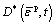.

Based on the above analysis, the rock salt dissolving model with mechanical effect can be set up as follow:

Assumed that the expression and assumption for the dissolving model without mechanical effect still can be applied to the dissolving model with mechanical effect.

Assumed that the new concept "equivalent diffusion coefficient" can be proposed to describe rock salt dissolving effect with mechanical effect.

constrained partial differentiation equation system of rock salt dissolving model with mechanical effect can be expressed as follow:(12)

In E. Q. 12, because the equivalent diffusion coefficient is the function byand t, the dissolving depth per unit area being computed can be called by equivalent dissolving depth R* which can be marked by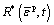.

According with E. Q. 12, if thevalue with different equivalent plastic strainand dissolving time t has been given, rock salt dissolving model with mechanical effect would be solved.

6. Calculation for Equivalent Diffusion Coefficient

Noticed that in E. Q. 12, thevalue with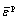and t can not be given by tests, but thevalue can be obtained by tests, so the computing way forcan be acquired as follow:

First, through tests thevalue has been obtained.

And then, thevalue has been substituted into the E. Q. 12, thevalue which is corresponding tovalue has been computed.

6.1. Test Datum

To acquire the rule of rock salt dissolving characteristics with mechanical effect, a series of rock salt uniaxial compression meso-mechanical tests under the coupled mechanical-dissolving effect have been tested.

The detail of test process and result has been shown in reference 14. The relationship between dissolved mass m and axial plastic strain εp and dissolving time t has been expressed as follow :(13)

In E. Q. 13, the unit of εp is %, 0εp8; the unit of t is 100s, 0t4.

6.2. Computing Way for D*

Under uniaxial compression, axial plastic strain can be used to describe the mechanical failure characteristics of rock salt, so based on the results of tests, εp can be used to replace equivalent plastic strainon the rock salt dissolving model with mechanical effect. If the D*(εp, t) value has been given, rock salt dissolving model with mechanical effect can be solved.

The computing way for D*(εp, t) has been shown as follow:

1)   Through analyzing the datum of tests, the relationship between equivalent dissolving depth R* and dissolved mass m can be expressed as follow: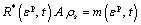(14)

In E. Q. 14, A is the surface area of rock salt sample being dissolved.

Jointed with E. Q. 13 and 14, the R* value can be obtained.

2)   And then by solved the E. Q. 12, the D*(εp, t) value with the certain value of εp and t can be computed.

6.3. Analysis for D*

6.3.1. Mathematical Model for D*

According with the test conditions of rock salt uniaxial compression meso-mechanical tests under the coupled mechanical-dissolving effect , the plane mathematical model for D* has been shown in Fig. 4.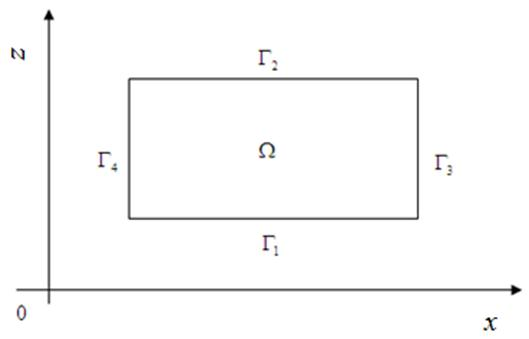Figure 4. Sketch of plane model for D*.

In Fig. 4, Γ1 is the surface of rock salt sample being dissolved; Γ2, Γ3 and Γ4 are free faces of the fluid, Γ2 is the surface of the fluid, Γ3 and Γ4 are the convective-diffusion faces that have been contacted with the surrounding fluid; Ω is the area for the fluid being modeled; in Ω, under the initial state, the fluid is stationary, the concentration C of fluid is a constant, and so E. Q. 8 and 9 can be used to describe the initial state of Mathematical model; z direction of the axis is the opposite direction of gravity.

The specific parameters for mathematical model have been shown as follow: ρw=1.0×103kg/m3, ρs=2.16×103kg/m3, M=6.88×10-2kg/mol, Cs=5.4×103mol/m3, v=1.14×10-6m2/s, C0=0, D=2.0×10-9m2/s.

6.3.2. Computing Process for D*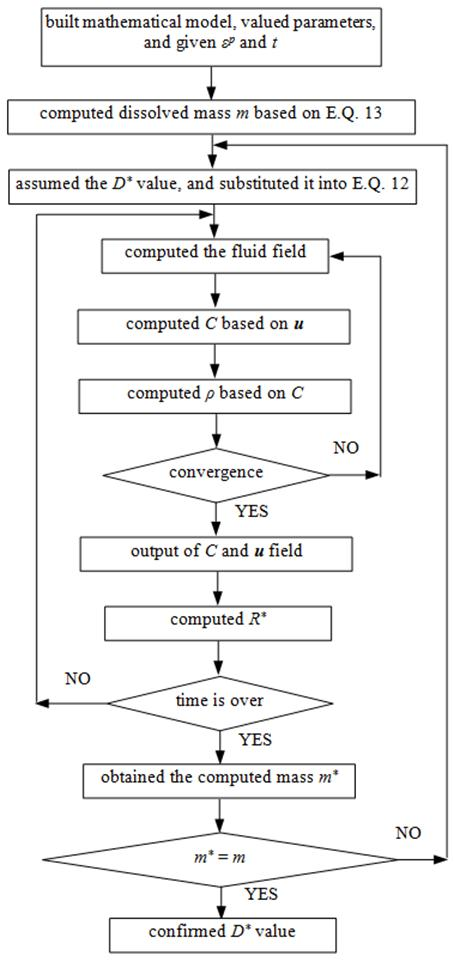Figure 5. Flow chart for computing D*.

The computing process for D* has been shown as follow:

Step 1: according with E. Q. 13, given the value of axial plastic strain εp and dissolving time t, the dissolved mass m can be computed.

Step 2: substituted the assumed value D* into E. Q. 12.

Step 3: according with E. Q. 12, the velocity, concentration and density of the fluid can be solved, and then equivalent dissolving depth R* can be obtained. Substituted the computed value of R* into E. Q. 14, computed mass m* can be obtained.

Step 4: compared with the computed mass m* and the dissolved mass m, if equally, D* with the given value of εp and t can be confirmed; otherwise, in the step 2 the assumed value of D* would be changed, the step 2, 3 and 4 would be executed again until the computed mass m* is equal to the dissolved mass m.

The flow chart for computing D* has been shown in Fig. 5. Noted that in Fig. 5 YES of "time is over" denotes that in solved E. Q 12 the time is equal to the given dissolving time t; otherwise, NO of "time is over" denotes that the time in solved E. Q 12 is less than the given t value.

6.3.3. Analysis for Computing Results

Through the above computing, the relationship between the value of D*(εp, t) divided by diffusion coefficient D (marked by D*/D) and axial plastic strain εp and dissolving time t has been expressed as follow:(15)

When the dissolving time t is 400 seconds, the relationship between D*/D and εp has been shown in Fig. 6. In Fig. 6, the curve has been expressed as follow: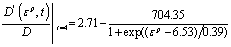(16)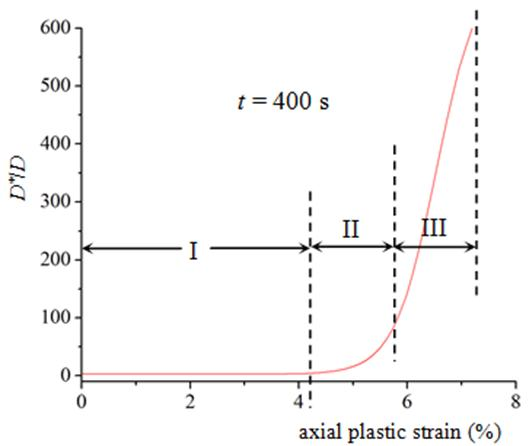Figure 6. Relationship between axial plastic strain and D*/D.

As shown in Fig. 6, according with the variation rule between D*/D and εp, the curve has been divided into three phases as follow:

Phase I

The curve of phase I is very gentle. On phase I, the value of D*/D is very small, and with εp increasing, the value of D*/D has been increased slowly, and the variation rate is very small.

The reason for the above phenomenon on phase I is that when the value of εp is smaller, the number of rock salt crack on the surface is less, during dissolving process, the dissolving action face has been varied faintly, and so the value of D* has been changed smaller.

Phase II

The curve of phase II is concave, and the rate of grade of the curve has been changed significantly. On phase II, with εp increasing, the value of D*/D has been increased obviously, and the rate of grade of D*/D is bigger.

The reason for the above phenomenon on phase II is that when εp has been attained a certain value, cracks on rock salt surface have been produced obviously, during dissolving process, the dissolving action face has been enlarged significantly, and then the value of D*/D has been increased obviously.

Phase III

On phase III, the rate of grade of the curve has been changed smaller, with εp increasing, the value of has been increased constantly. The reason for the above phenomenon is that on phase III with εp increasing, the dissolving action face has been enlarged continuously, the value of D* has been increased constantly but not obviously.

7. Conclusion

Rock salt dissolving mechanism with and without mechanical effect is same, but with mechanical effect, especially after the plastic strain and cracks have been produced, difference between the dissolving effect with and without mechanical effect has been existed. On this paper, through analyses on rock salt dissolving mechanism, and rock salt dissolving effect with and without mechanical effect, difference between rock salt dissolving model with and without mechanical effect has been studied. The conclusions have been shown as follow:

1)   The difference between rock salt dissolving effect with and without mechanical effect is that the dissolving action face has been changed. Without mechanical effect, dissolving action face is rock salt solid boundary surface, but with mechanical effect, dissolving action face has been enlarged that is the sum by the rock salt solid boundary surface and all surface cracks.

2)   With mechanical effect, the relationship has been existed in the macro-dissolving speed and plastic strain of rock salt.

3)   Compared with the parameter "diffusion coefficient" D on the dissolving model without mechanical effect, the new conception "equivalent diffusion coefficient" D* has been proposed to describe the macro-dissolving speed of rock salt with mechanical effect under the assumption that dissolving action face has not been changed during the dissolving process. D* is a variable of the equivalent plastic strain and dissolving time.

4)   Based on rock salt dissolving model without mechanical effect and the new concept D*, rock salt dissolving model with mechanical effect has been built.

5)   Through analyzing the datum of uniaxial compression meso-mechanical test under the coupled mechanical-dissolving effect, the method to calculate the equivalent diffusion coefficient D* has been proposed, and the relationship between axial plastic strain, dissolving time and D* has been obtained.

The achieved results of this paper can provide a foundation for the further analyses on coupled mechanical-dissolving mechanism of rock salt.

Acknowledgements

This paper is supported jointly by National Natural Science Foundation of China (51279089), and the open fund of State Key Laboratory of Geomechanics and Geotechnical Engineering (Institute of Rock and Soil Mechanics, Chinese Academy of Sciences) (Z012006).

References

1. TAN Yufei, CHEN Jiaxin, and YU Qizheng, "The Construction and Development of foreign underground gas storage caverns in salt formations" Oil & Gas Storage (in Chinese), vol. 20, n. 1, p. 6–8, 2001.
2. HE Jianying, ZHANG Dengqing, "Salt mine exploiting and petroleum reserve storage building" China Foreign Energy, vol. 11, n. 3, p. 7–11, 2006.
3. LI Jianzhong, "Using salt beds to build underground gas storages with salt Caves" Natural Gas Industry (in Chinese), vol. 24, n. 9, p. 119–121, 2004.
4. LIU Xinrong, JIANG Deyi, and XU Jiang, et al, "FEM analysis of stress distribution law in the rocksalt cavity’s surrounding rock" Journal of Chongqing University (in Chinese), vol. 26, n. 2, p. 39–41, 2003.
5. HOU Z, "Mechanical and hydraulic behavior of salt rock in the excavation disturbed zone around underground facilities" International Journal of Rock Mechanics and Mining Sciences, vol. 40, n. 5, p. 725–738, 2003.
6. Zhigalkin V. M., Usolfseva O. M., and Semenov V. N., et al, "Deformation of quasi-plastic salt rocks under different conditions of loading. Report I: Deformation of salt rocks under uniaxial compression" Journal of Mining Science, vol. 41, n. 6, p. 507–515, 2005.
7. Zhou H.W., Wang C. P., and Han B. B., et al, "A creep constitutive model for salt rock based on fractional derivatives" International Journal of Rock Mechanics and Mining Sciences, vol. 48, n. 1, p. 116–121, 2011.
8. YANG Junliu, YANG Jinchun, and ZOU Yushu, "The Study on Experiments of Solution Mining Properties of Salt" Journal of Sichuan Union University (Engineering Science Edition) (in Chinese), vol. 1, n. 2, p. 74–80, 1997.
9. LIANG Weiguo, "Study on multi-field coupling theory and its application of hydraulic fracturing and solution mining for salt deposit" Chinese Journal of Rock Mechanics and Engineering, vol. 24, n. 6, p. 1090–1090, 2005.
10. ZHOU Hui, TANG Yanchun, and HU Dawei, et al, "Study on coupled penetrating-dissolving model and experiment for salt rock cracks" Chinese Journal of Rock Mechanics and Engineering, vol. 25, n. 5, p. 946–950, 2006.
11. BAN Fansheng, GAO Shusheng, and SHAN Wenwen, "Rock salt grades influences on salt cavity gas storage built with water solution" Natural Gas Industry (in Chinese), vol. 26, n. 4, p. 115–118, 2006.
12. ZHAO Zhicheng, ZHU Weiyao, and SHAN Wenwen, et al, "Research on mechanism of solution mining for building underground gas storage in salt cavern" Petroleum Exploration and Development (in Chinese), vol. 30, n. 5, p. 107–109, 2003.
13. BAN Fansheng, GENG Jing, and GAO Shusheng, et al, "Studying on basic theory and influence factor of gas storage in Salt caverns building with water solution" Natural Gas Geoscience (in Chinese), vol. 17, n. 4, p. 261–266, 2006.
14. Tang Yanchun, Zhou Hui, and Feng Xiating, et al, "Analysis of Rock Salt Uniaxial Compression Meso-Mechanics Test Considering Coupled Stress-Dissolving Effect" Chinese Journal of Rock Mechanics and Engineering, vol. 27, n. 2, p. 294–302, 2008.

 Contents 1. 2. 3. 3.1. 3.2. 3.3. 3.4. 3.5. 3.6. 4. 5. 6. 6.1. 6.2. 6.3. 7.
Article ToolsAbstractPDF(382K)